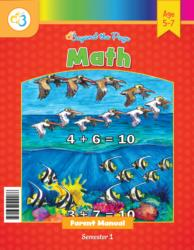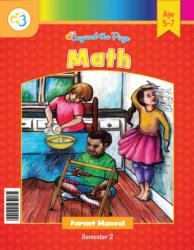# Common Core Alignment

CCSS.Math.Content.1.OA.3 - Apply properties of operations as strategies to add and subtract.3 Examples: If 8 + 3 = 11 is known, then 3 + 8 = 11 is also known. (Commutative property of addition.) To add 2 + 6 + 4, the second two numbers can be added to make a ten, so 2 + 6 + 4 = 2 + 10 = 12. (Associative property of addition.)

## 5: MathUnit 3: Addition and Subtraction to 10
Lesson 4: More Practice with AdditionUnit 5: Addition and Subtraction to 20